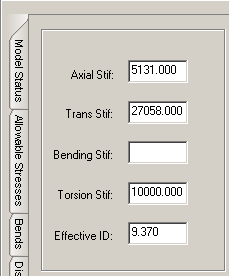# Simple Bellows with Pressure Thrust - CAESAR II - Reference Data

## CAESAR II Applications Guide (2019 Service Pack 1)

PPMProduct
CAESAR II
PPMCategory_custom
Reference Data
Version_CAESAR
11.0 (2019)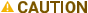The numbers used for the expansion joint examples in this topic are for illustrative purposes only. CAESAR II recommends that you verify all values used from a qualified technical source.

You can model bellows expansion joints using either a zero or a finite length. When finite length bellows are used, leave either the bending or the transverse stiffness blank. CAESAR II calculates the exact stiffness coefficient for the term left blank. If all stiffnesses are defined, CAESAR II uses the defined data and skips the stiffness calculations. For finite length expansion joints, leave the Bending Stif box empty and enter the lateral stiffness given by the manufacturer into the Trans Stif box on the Expansion Joints Auxiliary Data tab in the Classic Piping Input dialog box.

CAESAR II uses the Expansion Joint Manufacturer's Association (EJMA) equations to calculate the bending (angular) or transverse (lateral) stiffness of metallic bellows. EJMA defines these stiffness values as:

Ktr = (1.5Kax)(Deff/L)2 (1)

Kb = (Kax) (Deff)2/8 (2)

Where

Kb = Bending angular stiffness of the bellows

Ktr = Transverse lateral stiffness of the bellows

Kax = Axial stiffness of the bellows

Deff = Effective diameter mean diameter of the bellows

L = Length of the bellows for all convolutions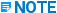Equation (2) above is based on modeling bellows as zero-length where the expansion joint acts as a hinge.

Because it is possible to leave the Effective ID box blank (zero), CAESAR II uses derived formulas for the calculation of the bending or transverse stiffness in terms of the other stiffness, thereby eliminating the effective diameter from the equations.

Kb = (KtrL2/3)(p/180) (3)Equation (3) has been increased by a factor of 4 from that derived from equations (1) and (2) above.

or

Ktr = (3Kb/L2)(180/p) (4)Equation (4) has been decreased by a factor of 4 from that derived from equations (1) and (2) above.

Bellows were originally modeled as zero-length elements. Finite-length bellows in bending cause a translation while zero-length bellows do not. For zero-length bellows, hold the translation of a finite-length bellows to zero while in bending. This requires a bending stiffness of four times that of the zero-length expansion joint, regardless of the length of the finite-length bellows. This is similar to the guided cantilever methods.

Most expansion joint manufacturer's catalog values for bending stiffness are still based on zero-length bellows, while the transverse stiffness is normally based on the finite-length of bellows. For more information refer to equations (1) and (2) above. For this reason, enter the transverse stiffness and leave the Bending Stif box blank. CAESAR II then calculates the proper bending stiffness according to equation (3) and uses this value for the flexibility analysis. If you enter a value for KTR that does not conform to equation (1), equation (3) uses the entered value to calculate Kb.If a catalog value of Kb is used and that value is based on zero-length values, CAESAR II uses equation (4), which results in one-quarter of the value of KTR.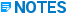• For a zero length expansion joint, CAESAR II uses either the preceding or the following element to determine the axial direction of the bellows stiffnesses. The preceding element is checked first.

• Bellows are very fragile under torsional loading. Accurate torsional stiffnesses and allowable torsional rotations should be obtained from the vendor.

• Systems using untied bellows should either be of very low pressure or adequately anchored and guided to withstand the possibly large thrust loads developed due to the unrestrained bellows.

• Bellows and any other miscellaneous weights should be added to flanges on either side of the bellows, or can be added as concentrated forces. This is particularly true when the bellow is part of a hanger sizing weight calculation.

A zero or blank Effective ID results in a zero pressure thrust. The Effective ID is the mean diameter of the bellows and is used to find the area for pressure thrust calculations. The total thrust load is applied at the From and To ends of the bellows and is used to open the bellows if the pressure is positive. The magnitude of the thrust load is P ´ Aeff, where P is the pressure in the pipe above atmospheric, and Aeff is the area, found from

Aeff = p/4 ´ (DEff)2

Many manufacturers specify the effective area of the bellows. The Effective ID for CAESAR II input can be calculated using the following equation:

Deff = [4Aeff /p]½

In the example below, the untied bellows runs between nodes 8 and 9. The elbow at node 11 is anchored to take the thrust load developed in the bellows. The manufacturer's specification for the axial stiffness of the joint is 5131 lbs/in. with a transverse stiffness of 27058 lbs/in. The bending stiffness is left blank and is calculated by CAESAR II because the bellows has a finite length. The pump and the baseplate at node 5 must be able to withstand the large axial force that develops due to pressure thrust in the bellows.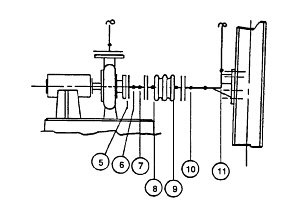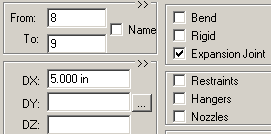Aeff = 69 in2 P = 175 psi Thrust = 69(175)=12075 lbs. (automatically applied by CAESAR II)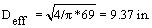* The bending stiffness (Bending Stif) calculated by CAESAR II: KBend = 1/3KtrL2(p/180) = 984 in-lbs/degree